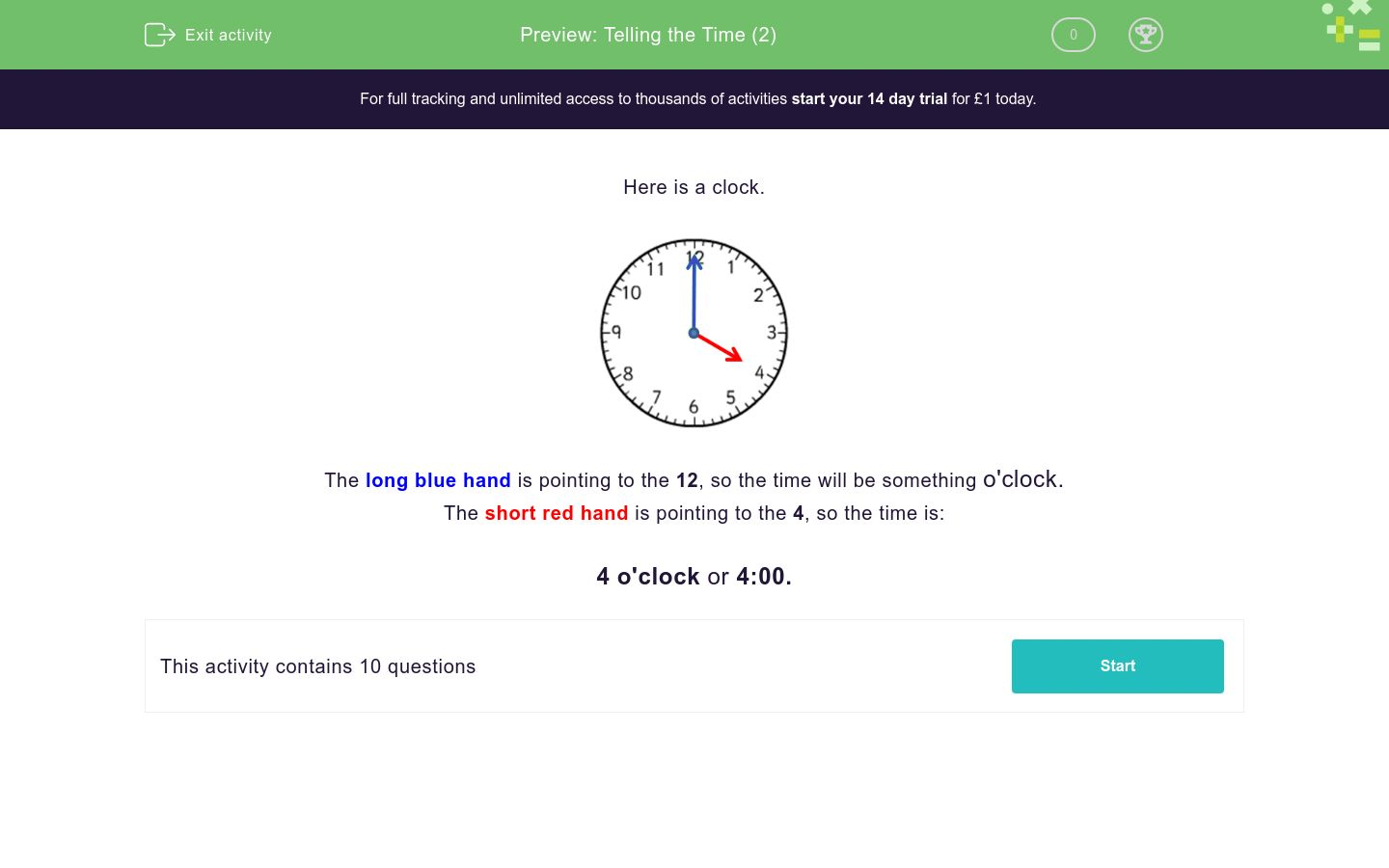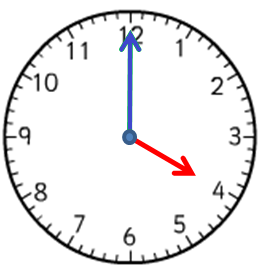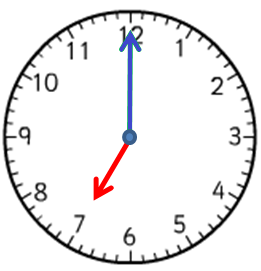# Telling the Time (2)

In this worksheet, students must select the clock face that matches the given time. Only o'clock times are shown.Key stage:  KS 1

Curriculum topic:   Measurement

Curriculum subtopic:   Tell the Time

Difficulty level:### QUESTION 1 of 10

Here is a clock.The long blue hand is pointing to the 12, so the time will be something o'clock.

The short red hand is pointing to the 4, so the time is:

4 o'clock or 4:00.

The time is

7 o'clock.

Which clock face shows this time?A B C
A

B

C

The time is

5 o'clock.

Which clock face shows this time?A B C
A

B

C

The time is

3 o'clock.

Which clock face shows this time?A B C
A

B

C

The time is

2:00 o'clock.

Which clock face shows this time?A B C
A

B

C

The time is

10:00 o'clock.

Which clock face shows this time?A B C
A

B

C

The time is

4:00 o'clock.

Which clock face shows this time?A B C
A

B

C

The time is

1:00 o'clock.

Which clock face shows this time?A B C
A

B

C

The time is

9:00 o'clock.

Which clock face shows this time?A B C
A

B

C

The time is

6 o'clock.

Which clock face shows this time?A B C
A

B

C

The time is

12 o'clock.

Which clock face shows this time?A B C
A

B

C

• Question 1

The time is

7 o'clock.

Which clock face shows this time?A B C
A
EDDIE SAYS
The short hand is pointing to 7.
The long hand is pointing to 12.
• Question 2

The time is

5 o'clock.

Which clock face shows this time?A B C
C
EDDIE SAYS
The short hand is pointing to 5.
The long hand is pointing to 12.
• Question 3

The time is

3 o'clock.

Which clock face shows this time?A B C
B
EDDIE SAYS
The short hand is pointing to 3.
The long hand is pointing to 12.
• Question 4

The time is

2:00 o'clock.

Which clock face shows this time?A B C
A
EDDIE SAYS
The short hand is pointing to 2.
The long hand is pointing to 12.
• Question 5

The time is

10:00 o'clock.

Which clock face shows this time?A B C
C
EDDIE SAYS
The short hand is pointing to 10.
The long hand is pointing to 12.
• Question 6

The time is

4:00 o'clock.

Which clock face shows this time?A B C
B
EDDIE SAYS
The short hand is pointing to 4.
The long hand is pointing to 12.
• Question 7

The time is

1:00 o'clock.

Which clock face shows this time?A B C
A
EDDIE SAYS
The short hand is pointing to 1.
The long hand is pointing to 12.
• Question 8

The time is

9:00 o'clock.

Which clock face shows this time?A B C
C
EDDIE SAYS
The short hand is pointing to 9.
The long hand is pointing to 12.
• Question 9

The time is

6 o'clock.

Which clock face shows this time?A B C
B
EDDIE SAYS
The short hand is pointing to 6.
The long hand is pointing to 12.
• Question 10

The time is

12 o'clock.

Which clock face shows this time?A B C
C
EDDIE SAYS
The short hand is pointing to 12.
The long hand is pointing to 12.
---- OR ----

Sign up for a £1 trial so you can track and measure your child's progress on this activity.

### What is EdPlace?

We're your National Curriculum aligned online education content provider helping each child succeed in English, maths and science from year 1 to GCSE. With an EdPlace account you’ll be able to track and measure progress, helping each child achieve their best. We build confidence and attainment by personalising each child’s learning at a level that suits them.

Get started